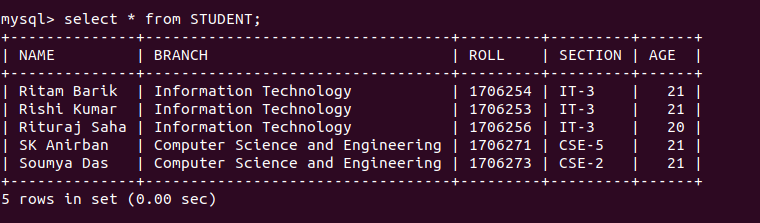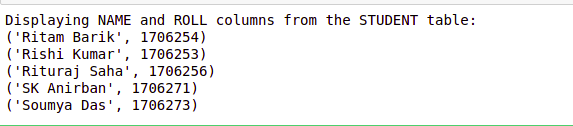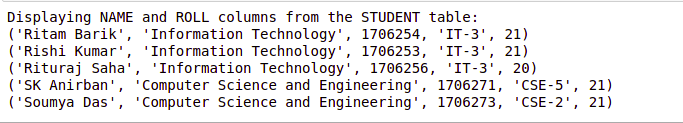Open In App
Related Articles

# Python MySQL – Select Query

Python Database API ( Application Program Interface ) is the Database interface for the standard Python. This standard is adhered to by most Python Database interfaces. There are various Database servers supported by Python Database such as MySQL, GadFly, mySQL, PostgreSQL, Microsoft SQL Server 2000, Informix, Interbase, Oracle, Sybase etc. To connect with MySQL database server from Python, we need to import the mysql.connector module. Below is a program to connect with MySQL database geeks.

## Python

 `# importing required library``import` `mysql.connector` `# connecting to the database``dataBase ``=` `mysql.connector.connect(``                     ``host ``=` `"localhost",``                     ``user ``=` `"user",``                     ``passwd ``=` `"pswrd",``                     ``database ``=` `"geeks" )` `# preparing a cursor object``cursorObject ``=` `dataBase.cursor()` `# disconnecting from server``dataBase.close()`

The above program illustrates the connection with the MySQL database geeks in which host-name is localhost, the username is user and password is pswrd.

## Select Query

After connecting with the database in MySQL we can select queries from the tables in it. Syntax:

• In order to select particular attribute columns from a table, we write the attribute names.
`SELECT attr1, attr2 FROM table_name`
• In order to select all the attribute columns from a table, we use the asterisk ‘*’ symbol.
`SELECT * FROM table_name`

Example 1: Let’s consider the table looks like this –Below is a program to select a query from the table in the database.

## Python

 `# importing required library``import` `mysql.connector``  ` `# connecting to the database``dataBase ``=` `mysql.connector.connect(``                     ``host ``=` `"localhost",``                     ``user ``=` `"user",``                     ``passwd ``=` `"pswrd",``                     ``database ``=` `"geeks" )``  ` `# preparing a cursor object``cursorObject ``=` `dataBase.cursor()``  ` `print``("Displaying NAME ``and` `ROLL columns ``from` `the STUDENT table:")` `# selecting query``query ``=` `"SELECT NAME, ROLL FROM STUDENT"``cursorObject.execute(query)` `myresult ``=` `cursorObject.fetchall()` `for` `x ``in` `myresult:``    ``print``(x)` `# disconnecting from server``dataBase.close()`

Output:Example 2: Let us look at another example for selecting queries in a table.

## Python

 `# importing required library``import` `mysql.connector``  ` `# connecting to the database``dataBase ``=` `mysql.connector.connect(``                     ``host ``=` `"localhost",``                     ``user ``=` `"user",``                     ``passwd ``=` `"pswrd",``                     ``database ``=` `"geeks" ) ``  ` `# preparing a cursor object``cursorObject ``=` `dataBase.cursor()``  ` `print``("Displaying NAME ``and` `ROLL columns ``from` `the STUDENT table:")` `# selecting query``query ``=` `"SELECT ``*` `FROM STUDENT"``cursorObject.execute(query)` `myresult ``=` `cursorObject.fetchall()` `for` `x ``in` `myresult:``    ``print``(x)` `# disconnecting from server``dataBase.close()`

Output: